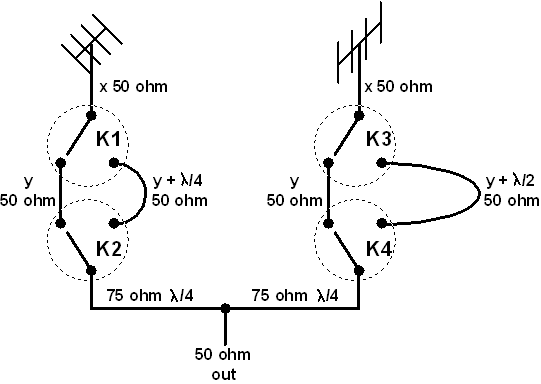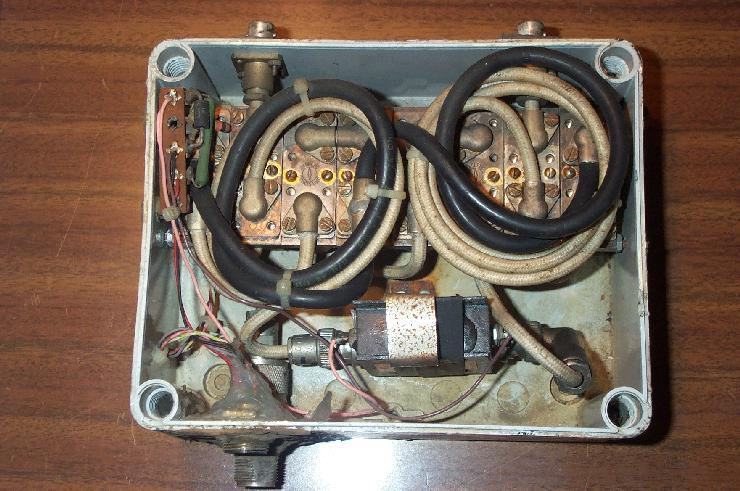Hi, I am Tony I0JX
An
antenna system with four polarizations, linear and circular

At VHF and UHF, hams utilize both linear and circular polarization:

• linear polarization (horizontal, vertical) is used for normal direct communications;
• circular polarization (left-hand, right-hand) has been introduced with the advent of amateur radio satellites (Oscar).

Almost twenty years ago I decided to build an antenna system for both 2 meters and 70 centimeters having the possibility to generate four polarizations, i.e. horizontal, vertical, right-hand and left-hand circular. The system was built around:

• one Tonna 11+11-element crossed Yagi for 144 MHz (mod. 20822). This actually comprises two fully independent antennas with the orthogonal elements mounted on the same boom. The boom-to-mast clamp is designed in such a way that all elements are at 45 degrees with respect to the support mast;
• two Tonna 21-element Yagis (mod. 20921) for 430 MHz mounted on a V-shaped mechanical adapter. The elements of two antennas are orthogonal and they all are at 45 degrees with respect to the support legs.To obtain the four polarizations I adopted the following circuit which utilizes four SPDT coaxial relays (K1 through K4).The picture shows the length and the impedance of the various coaxial cable sections.

The two cable sections joining the antennas to relays K1 to K3 must be 50 ohm and have exactly the same length (marked "x"), though arbitrary.

The four sections joining relay K1 to K2, or relay K3 to K4, must all be 50 ohm and have a length that is either "y" (an arbitrary value) or "y" plus a given fraction of the wavelength (one half or one fourth). Clearly "y", once chosen, shall be identical for the four sections.

Two 75-ohm quarter-wavelength sections provide for the required impedance transformation before the two antennas are put in parallel (i.e. at the signals summation point). So the system output impedance remains 50 ohm.

Remember to take into account the cable velocity factor when cutting the various cable sections. .

The working principle is so explained:

• when no relay is energized, the total paths length from the antenna to the summation point are identical for the two antennas. So the two antenna signals add in phase, and the resulting polarization is then linear. Whether vertical or horizontal, this will depend on the phase by which the antenna radiators are fed (phase depends on which of the two antenna terminals the coaxial cable inner conductor is attached to);
• if K3 and K4 are energized, there will be an half-wavelength shift between the two paths, this causing the polarization to shift by 180 degrees, i.e. it will remain linear but orthogonal to the previous case;
• if K1 and K2 are instead energized, the shift between the two paths will become one-quarter wavelength, or 90 degrees, this resulting into circular polarization (whether left-hand or right-hand again depends on the antenna radiators feed phase);
• if all four relays are energized, the opposite circular polarization will be produced.

I used a total of eigth coaxial relays, four for polarization switching (as discussed above) and four for switching in a preamplifier and grounding its terminals when not in use. It is important to use relays of the solder type, i.e. having no connectors, otherwise the system would become too bulky and losses would increase.

Below you can see the 144-MHz box with eigth coaxial relays mounted neck-to-neck in a water-proof box. You can see that this arrangement results in the shortest possible connections. The 430 MHz box is similar, apart from the shorter coaxial cable sections length.50-ohm cable is teflon RG-141 (velocity factor: 0.695), while 75-ohm cable is RG-59 (velocity factor: 0.659).

All cable sections must be cut very precisely, especially those of the 430 MHz unit. The box shall be located fairly close to the antenna, so as to make the length marked "x" as short as possible (so reducing the inevitable errors when cutting lengths).

When the antenna elements are on the same boom (like the Tonna mod. 20822), the two radiators are inevitably offset by a few centimeters. Such mechanical shift must be compensated for by varying the length "x" on just one of the two paths (also in this case remember to take into account the cable velocity factor).

The results were outstanding, as the obtained polarization purity was very good for both the 144 MHz and the 430 MHz antennas.